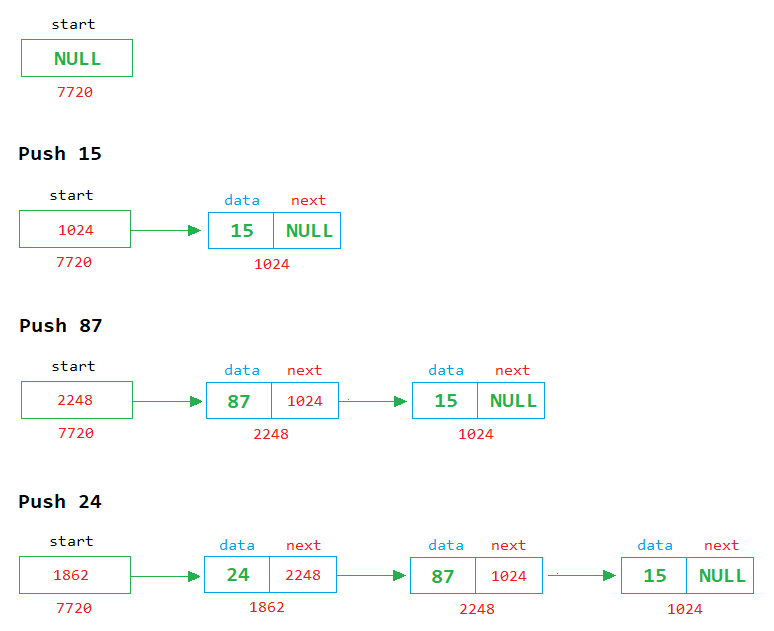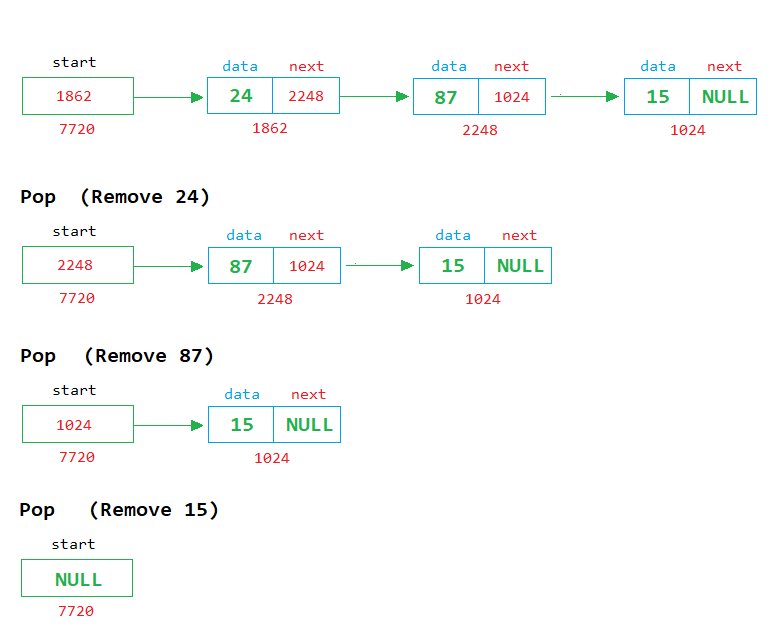# Stack using Linked List in C++

###### Data Structure in C++

In this lesson, we will learn how to implement the stack using a singly linked list.

## Linked List Implementation of Stack in C++

We know about the stack and how to implement it using an array. In this lesson, we will learn how to implement the stack using a singly linked list.

We also know that there are two operations possible on the stack, push and pop. See the image given below to clearly understand how to implement push and pop operation on a stack using a linked list.

### Push ExampleIn the above image, we can see that when a new node is created, it is placed in front of the first node and its address is stored in the start pointer.

### Pop ExampleIn the above image, we can see that each time when a node is removed, the first node is deleted from the list and the address of the next node is stored in the start pointer.## Program of Stack using Linked List

Below is the complete program of stack in C++ using singly linked list having size 5.

#### Stack Program in C++ using Singly Linked List

``````#include <iostream>
#include <conio.h>
#include <stdlib.h>
#include <malloc.h>

using namespace std;

#define size 5

// Define node structure
typedef struct node
{
int data;
struct node *next;
} node;

int main()
{
node *start=NULL,*temp;
int top=0,ch,n;

for(;;)		// An infinite loop
{
system("cls");		// for clearing the screen
cout<<"1. Push\n";
cout<<"2. Pop\n";
cout<<"3. Display\n";
cout<<"4. Exit\n";
cout<<"Enter Choice: ";
cin>>ch;

switch(ch)
{
case 1:
if(top==size)
{
cout<<"Stack is full";
getch();	// pause the loop to see the message
}
else
{
cout<<"Enter a number ";
cin>>n;

//Create a new node
temp=new node;
temp->data=n;
temp->next=NULL;

if(start==NULL)
{
start=temp;
}
else
{
// insert the new node before the first node
temp->next=start;
start=temp;
}
top++;
}
break;

case 2:
if(start==NULL)
{
cout<<"Stack is empty";
getch();	// pause the loop to see the message
}
else
{
cout<<"Number Popped = "<<start->data;
temp=start;
start=start->next;
delete temp;
top--;
getch();	// pause the loop to see the number
}
break;

case 3:
if(start==NULL)
{
cout<<"Stack is empty";
getch();	// pause the loop to see the message
}
else
{
temp=start;		// start from 1st node

// display the nodes on the screen
while(temp!=NULL)
{
cout<<temp->data<<endl;
temp=temp->next;
}
getch(); // pause the loop to see the nodes
}
break;

case 4:
exit(0);
break;

default:
cout<<"Wrong Choice";
getch();	// pause the loop to see the message
}
}
return 0;
}``````